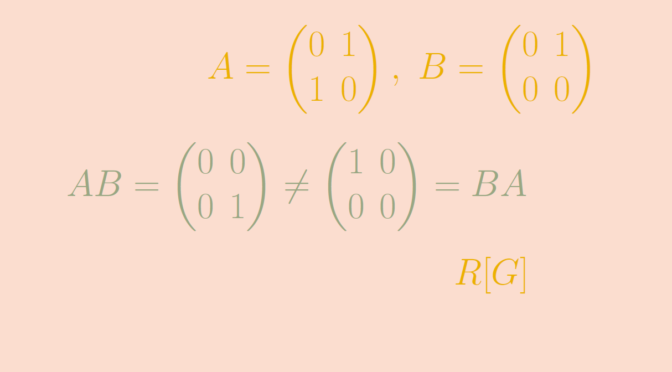# Non commutative rings

Let’s recall that a set $$R$$ equipped with two operations $$(R,+,\cdot)$$ is a ring if and only if $$(R,+)$$ is an abelian group, multiplication $$\cdot$$ is associative and has a multiplicative identity $$1$$ and multiplication is left and right distributive with respect to addition.

$$(\mathbb Z, +, \cdot)$$ is a well known infinite ring which is commutative. The rational, real and complex numbers are other infinite commutative rings. Those are in fact fields as every non-zero element have a multiplicative inverse.

For a field $$F$$ (finite or infinite), the polynomial ring $$F[X]$$ is another example of infinite commutative ring.

Also for $$n$$ integer, the integers modulo n is a finite ring that is commutative. Finally, according to Wedderburn theorem every finite division ring is commutative.

So what are examples of non commutative rings? Let’s provide a couple.

### Matrix rings

Consider a field $$F$$ and an integer $$n \ge 2$$. The set $$M_n(F)$$ of square matrices over the field $$F$$ is a ring. $$M_n(F)$$ is never commutative. Indeed, given the matrices $A=\begin{pmatrix} 0 & 1 \\ 1 & 0 \end{pmatrix}, \ B=\begin{pmatrix} 0 & 1 \\ 0 & 0 \end{pmatrix},$ we have $AB= \begin{pmatrix} 0 & 0 \\ 0 & 1 \end{pmatrix} \neq \begin{pmatrix} 1 & 0 \\ 0 & 0 \end{pmatrix} = BA.$

Therefore with matrix rings we get examples of non-commutative rings that can be finite or infinite depending on whether $$F$$ is finite or not. One can notice that $$M_n(F)$$ is never a division ring as $$B^2=0$$. Consequently $$B$$ cannot have a multiplicative inverse.

### Group rings

Considering a group $$G$$ and a ring $$R$$. The group ring of $$G$$ over $$R$$, denoted by $$R[G]$$ is the set of mappings $$f : G \to R$$ with finite support, i.e. taking a finite number of non-zero values. The addition of two elements $$f,g \in R[G]$$ is the mapping $$f+g: x \mapsto f(x)+g(x)$$. The product is the mapping $f \cdot g : x \mapsto \sum_{uv=x} f(u) g(v) = \sum_{u \in G} f(u)g(u^{-1}x)$ which is well defined because $$f,g$$ are of finite support.

One can verify that $$R[G]$$ is indeed a ring.

When $$G$$ is a non-commutative group, $$R[G]$$ is a non-commutative ring as for $$r,s \in G$$ such that $$rs \neq sr$$ we have $$\mathbf 1_{rs} \neq \mathbf 1_{sr}$$. Where for $$g \in G$$, $$\mathbf 1_{g} \in R[G]$$ denotes the finite support function that maps $$g$$ to $$1$$ and all the other elements to $$0$$.